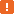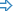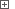# Accessing Rows, Columns and Cells in Syncfusion GridDataBoundGridIn order to perform various operations over Syncfusion's GridDataBoundGrid control, you will need to obtain the objects corresponding to grid rows, columns and cells. This topic describes the properties and methods of the GridDataBoundGrid object that can be used for this purpose.To perform these actions, TestComplete should have access to internal objects, properties and methods of the GridDataBoundGrid control. For this purpose, the .NET Application Support plugin must be installed and enabled.When testing Syncfusion GridDataBoundGrid controls, use specific methods and properties of the corresponding `SyncfusionEssGrid` object. You can call these methods and properties from your keyword tests, as well as from scripts. This topic describes how to work with an object’s properties and methods from your scripts. However, when testing a GridDataBoundGrid control from your keyword test, you can use the same methods and properties calling them from keyword test operations. For more information, see Keyword Tests Basic Operations.
##### Identifying Rows and Columns

The rows and columns in the GridDataBoundGrid control are identified by their indexes. The numerations are zero-based, so the first row and column have an index of 0, the second - 1, and so on. The index of the last grid row is `Model.RowCount` and last column is `Model.ColCount`. So, the total number of grid rows is `Model.RowCount`+1 and for columns, `Model.ColCount`+1.

Note that the rows and columns numeration includes row and column headers. That is, the starting indexes correspond to grid headers and indexes of the actual data rows and columns start from 1 or a greater number, depending on the number of headers. If you need to locate the range of actual data cells, use the grid’s `GridCellsRange` property. The `Top`, `Bottom`, `Left` and `Right` properties of the returned object let you get bound indexes of grid rows and columns that contain data cells. To determine the number of data rows and columns, use the `GridCellsRange.Height` and `GridCellsRange.Width` properties, respectively.

The following example works with the GDBGMultiHeader sample application that comes with Syncfusion's Essential Studio library and resides in the following folder.<Syncfusion>\Windows\Grid.Windows\Samples\2.0\DataBound\GDBG Multi Header Demo\

JavaScript, JScript

function Test ()
{
var p, Grid;

// Obtain the application process and the grid object

// Post information about the grid structure to the test log
Log.Message ("Total number of rows: " + (Grid.Model.RowCount+1));
Log.Message ("Total number of columns: " + (Grid.Model.ColCount+1));
Log.Message ("Number of data rows: " + Grid.GridCellsRange.Height);
Log.Message ("Number of data columns: " + Grid.GridCellsRange.Width);
Log.Message ("Index of the first data row: " + Grid.GridCellsRange.Top);
Log.Message ("Index of the first data column: " + Grid.GridCellsRange.Left);
}

Python

``````def Test ():

# Obtain the application process and the grid object

# Post information about the grid structure to the test log
Log.Message ("Total number of rows: " + (Grid.Model.RowCount+1));
Log.Message ("Total number of columns: " + (Grid.Model.ColCount+1));
Log.Message ("Number of data rows: " + Grid.GridCellsRange.Height);
Log.Message ("Number of data columns: " + Grid.GridCellsRange.Width);
Log.Message ("Index of the first data row: " + Grid.GridCellsRange.Top);
Log.Message ("Index of the first data column: " + Grid.GridCellsRange.Left);``````

VBScript

Sub Test
Dim p, Grid

' Obtain the application process and the grid object

' Post information about the grid structure to the test log
Call Log.Message ("Total number of rows: " & (Grid.Model.RowCount+1))
Call Log.Message ("Total number of columns: " & (Grid.Model.ColCount+1))
Call Log.Message ("Number of data rows: " & Grid.GridCellsRange.Height)
Call Log.Message ("Number of data columns: " & Grid.GridCellsRange.Width)
Call Log.Message ("Index of the first data row: " & Grid.GridCellsRange.Top)
Call Log.Message ("Index of the first data column: " & Grid.GridCellsRange.Left)
End Sub

DelphiScript

procedure Test;
var p, Grid : OleVariant;
begin
// Obtain the application process and the grid object

// Post information about the grid structure to the test log
Log.Message ('Total number of rows: ' + aqConvert.VarToStr(Grid.Model.RowCount+1));
Log.Message ('Total number of columns: ' + aqConvert.VarToStr(Grid.Model.ColCount+1));
Log.Message ('Number of data rows: ' + aqConvert.VarToStr(Grid.GridCellsRange.Height));
Log.Message ('Number of data columns: ' + aqConvert.VarToStr(Grid.GridCellsRange.Width));
Log.Message ('Index of the first data row: ' + aqConvert.VarToStr(Grid.GridCellsRange.Top));
Log.Message ('Index of the first data column: ' + aqConvert.VarToStr(Grid.GridCellsRange.Left));
end;

C++Script, C#Script

function Test ()
{
var p, Grid;

// Obtain the application process and the grid object

// Post information about the grid structure to the test log
Log["Message"]("Total number of rows: " + (Grid["Model"]["RowCount"]+1));
Log["Message"]("Total number of columns: " + (Grid["Model"]["ColCount"]+1));
Log["Message"]("Number of data rows: " + Grid["GridCellsRange"]["Height"]);
Log["Message"]("Number of data columns: " + Grid["GridCellsRange"]["Width"]);
Log["Message"]("Index of the first data row: " + Grid["GridCellsRange"]["Top"]);
Log["Message"]("Index of the first data column: " + Grid["GridCellsRange"]["Left"]);
}

##### Accessing Cells

To access a particular cell in the GridDataBoundGrid control, use the grid’s `Item` property that takes the desired cell’s row and column indexes as parameters. However, sometimes it may be more useful to access cells specifies by column names rather than indexes. Since the order of columns in the GridDataBoundGrid control can be customized, column names, which are defined in the application code, can be more reliable that indexes. If you decide to refer to columns by their names, you will additionally need to use the grid’s `ColNameToIndex` method to get the column’s index by its name.

To obtain information about the currently selected cell, use the grid’s `CurrentCell` property. The `CurrentCell.RowIndex` and `CurrentCell.ColIndex` properties let you determine indexes of the current row and column, respectively.

The code snippet below demonstrates how you can get information about the currently selected grid cell:

###### ExampleView description

JavaScript

function Main ()
{
var p, Grid, Cell;

// Obtain the application process and the grid object

// Click a grid cell
ClickCell (Grid, 11, "CompanyName");

// Get information about the selected cell
Cell = Grid.CurrentCell;
Log.Message ("Current row index: " + Cell.RowIndex);
Log.Message ("Current column index: " + Cell.ColIndex);
Log.Message ("Current cell text: " + Grid.Item(Cell.RowIndex, Cell.ColIndex).FormattedText.OleValue);
}

function ClickCell (Grid, RowIndex, ColumnId)
{
// Convert the column's identifier to its absolute index
let ColIndex = GetColIndexById (Grid, ColumnId);
// Make cell visible
Grid.ScrollCellInView_3 (RowIndex, ColIndex);
// Get the cell coordinates
let rect = Grid.RangeInfoToRectangle (Grid.GridCellsRange.Cell(RowIndex, ColIndex));

Grid.Click (rect.X + rect.Width/2, rect.Y + rect.Height/2);
}

function GetColIndexById (Grid, ColumnId)
{
// Check if the column is specified by name or index
if (equal(aqObject.GetVarType(ColumnId), varOleStr))
// Get the column index by name
return Grid.NameToColIndex(ColumnId)
else
// Return the column index unchanged
return ColumnId;
}

JScript

function Main ()
{
var p, Grid, Cell;

// Obtain the application process and the grid object

// Click a grid cell
ClickCell (Grid, 11, "CompanyName");

// Get information about the selected cell
Cell = Grid.CurrentCell;
Log.Message ("Current row index: " + Cell.RowIndex);
Log.Message ("Current column index: " + Cell.ColIndex);
Log.Message ("Current cell text: " + Grid.Item(Cell.RowIndex, Cell.ColIndex).FormattedText.OleValue);
}

function ClickCell (Grid, RowIndex, ColumnId)
{
// Convert the column's identifier to its absolute index
var ColIndex = GetColIndexById (Grid, ColumnId);
// Make cell visible
Grid.ScrollCellInView_3 (RowIndex, ColIndex);
// Get the cell coordinates
var rect = Grid.RangeInfoToRectangle (Grid.GridCellsRange.Cell(RowIndex, ColIndex));

Grid.Click (rect.X + rect.Width/2, rect.Y + rect.Height/2);
}

function GetColIndexById (Grid, ColumnId)
{
// Check if the column is specified by name or index
if (aqObject.GetVarType(ColumnId) == varOleStr)
// Get the column index by name
return Grid.NameToColIndex(ColumnId)
else
// Return the column index unchanged
return ColumnId;
}

Python

``````def Main ():

# Obtain the application process and the grid object

# Click a grid cell
ClickCell (Grid, 11, "CompanyName");

# Get information about the selected cell
Cell = Grid.CurrentCell;
Log.Message ("Current row index: " + Cell.RowIndex);
Log.Message ("Current column index: " + Cell.ColIndex);
Log.Message ("Current cell text: " + Grid.Item(Cell.RowIndex, Cell.ColIndex).FormattedText.OleValue);

def ClickCell (Grid, RowIndex, ColumnId):
# Convert the column's identifier to its absolute index
ColIndex = GetColIndexById (Grid, ColumnId);
# Make cell visible
Grid.ScrollCellInView_3 (RowIndex, ColIndex);
# Get the cell coordinates
rect = Grid.RangeInfoToRectangle (Grid.GridCellsRange.Cell(RowIndex, ColIndex));

Grid.Click (rect.X + rect.Width/2, rect.Y + rect.Height/2);

def GetColIndexById (Grid, ColumnId):
# Check if the column is specified by name or index
if (aqObject.GetVarType(ColumnId) == varOleStr):
# Get the column index by name
return Grid.NameToColIndex(ColumnId)
else:
# Return the column index unchanged
return ColumnId;``````

VBScript

Sub Main
Dim p, Grid, Cell

' Obtain the application process and the grid object

' Click a grid cell
Call ClickCell (Grid, 11, "CompanyName")

' Get information about the selected cell
Set Cell = Grid.CurrentCell
Log.Message ("Current row index: " & Cell.RowIndex)
Log.Message ("Current column index: " & Cell.ColIndex)
Log.Message ("Current cell text: " & Grid.Item(Cell.RowIndex, Cell.ColIndex).FormattedText.OleValue)
End Sub

Sub ClickCell (Grid, RowIndex, ColumnId)
Dim ColIndex, rect

' Convert the column's identifier to its index
ColIndex = GetColIndexById (Grid, ColumnId)

' Make cell visible
Call Grid.ScrollCellInView_3 (RowIndex, ColIndex)

' Get the cell coordinates
Set rect = Grid.RangeInfoToRectangle (Grid.GridCellsRange.Cell(RowIndex, ColIndex))

Call Grid.Click (rect.X + rect.Width/2, rect.Y + rect.Height/2)
End Sub

Function GetColIndexById (Grid, ColumnId)
' Check if the column is specified by name or index
If aqObject.GetVarType(ColumnId) = varOleStr Then
' Get the column index by name
GetColIndexById = Grid.NameToColIndex(ColumnId)
Else
' Return the column index unchanged
GetColIndexById = ColumnId
End If
End Function

DelphiScript

procedure ClickCell (Grid, RowIndex, ColumnId); forward;
function GetColIndexById (Grid, ColumnId); forward;

procedure Main;
var p, Grid, Cell : OleVariant;
begin
// Obtain the application process and the grid object

// Click a grid cell
ClickCell (Grid, 11, 'CompanyName');

// Get information about the selected cell
Cell := Grid.CurrentCell;
Log.Message ('Current row index: ' + aqConvert.VarToStr(Cell.RowIndex));
Log.Message ('Current column index: ' + aqConvert.VarToStr(Cell.ColIndex));
Log.Message ('Current cell text: ' + Grid.Item[Cell.RowIndex, Cell.ColIndex].FormattedText.OleValue);
end;

procedure ClickCell (Grid, RowIndex, ColumnId);
var ColIndex, rect : OleVariant;
begin
// Convert the column's identifier to its index
ColIndex := GetColIndexById (Grid, ColumnId);

// Make cell visible
Grid.ScrollCellInView_3 (RowIndex, ColIndex);

// Get the cell coordinates
rect := Grid.RangeInfoToRectangle (Grid.GridCellsRange.Cell(RowIndex, ColIndex));

Grid.Click (rect.X + rect.Width/2, rect.Y + rect.Height/2);
end;

function GetColIndexById (Grid, ColumnId);
begin
// Check if the column is specified by name or index
if aqObject.GetVarType(ColumnId) = varOleStr then
// Get the column index by name
Result := Grid.NameToColIndex(ColumnId)
else
// Return the column index unchanged
Result := ColumnId;
end;

C++Script, C#Script

function Main ()
{
var p, Grid, Cell;

// Obtain the application process and the grid object

// Click a grid cell
ClickCell (Grid, 11, "CompanyName");

// Get information about the selected cell
Cell = Grid["CurrentCell"];
Log["Message"]("Current row index: " + Cell["RowIndex"]);
Log["Message"]("Current column index: " + Cell["ColIndex"]);
Log["Message"]("Current cell text: " + Grid["Item"](Cell["RowIndex"], Cell["ColIndex"])["FormattedText"]["OleValue"]);
}

function ClickCell (Grid, RowIndex, ColumnId)
{
// Convert the column's identifier to its absolute index
var ColIndex = GetColIndexById (Grid, ColumnId);
// Make cell visible
Grid["ScrollCellInView_3"](RowIndex, ColIndex);
// Get the cell coordinates
var rect = Grid["RangeInfoToRectangle"](Grid["GridCellsRange"]["Cell"](RowIndex, ColIndex));

Grid["Click"](rect["X"] + rect["Width"]/2, rect["Y"] + rect["Height"]/2);
}

function GetColIndexById (Grid, ColumnId)
{
// Check if the column is specified by name or index
if (aqObject["GetVarType"](ColumnId) == varOleStr)
// Get the column index by name
return Grid["NameToColIndex"](ColumnId)
else
// Return the column index unchanged
return ColumnId;
}